# Texas Go Math Grade 2 Lesson 1.3 Answer Key Explore 3-Digit Numbers

Refer to our Texas Go Math Grade 2 Answer Key Pdf to score good marks in the exams. Test yourself by practicing the problems from Texas Go Math Grade 2 Lesson 1.3 Answer Key Using Explore 3-Digit Numbers.

## Texas Go Math Grade 2 Lesson 1.3 Answer Key Explore 3-Digit Numbers

Explore

Draw to model the problem. Write the numbers.
Mrs Rodriguez
__________ straws
Mrs Davis
__________ straws

FOR THE TEACHER • Read the following problem. Mrs. Rodriguez has one box of 100 straws. Mrs. Davis has 10 boxes of 10 straws. How many straws do they each have?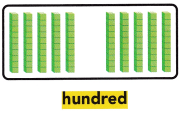Mrs Rodriguez 100 straws.
Mrs Davis 100 straws.
He has 10 boxes of 10 straws means each box is having 10 straws and 10 boxes are there. So totally he has 10*10=100 straws.

Math Talk
Mathematical Processes

Describe how you can compare 100 to 10 tens.
10 tens are nothing but 100In each stack, 10 blocks are there. Here 10 tens are there.
If we count all those 10’s we get 100.
so easily we can recognize by using stacks.

Model and Draw

What number is shown with 11 tens?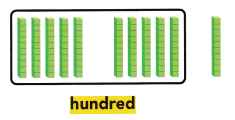___________ tens = ___________
___________ hundred __________ ten
___________ + ___________
In the number 110, there is a 1 in the hundreds place and a 1 in the tens place.
11 tens=110
1 hundred 10 tens
100+10
The number of blocks in each stack=10
The number of stacks in the row=100
10 tens=100
The number of stacks extra=1
1 tens=10
Total: 100+10=110
We can write numbers in different ways such as place value, expanded form, word form and standard form:
The standard form of 11 tens is 110
The expanded form of 110 is 100+10+0
The word form of 110 is one hundred ten.
The place value of 110 is 0 is in one’s place, 1 is in the tens place, 1 is in the hundreds place.

Share and Show

Circle tens to make 1 hundred. Write the number in different ways.

Question 1.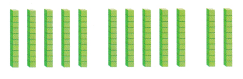___________ tens = ___________
___________ hundred __________ ten
___________ + ___________
12 tens=120
1 hundred 20 ten
100+20
Explanation: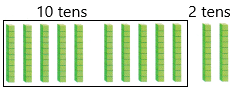The number of blocks in each stack=10
The number of stacks in the row=100
10 tens=100
The number of stacks extra=2
2 tens=20
Total: 100+20=120
We can write numbers in different ways such as place value, expanded form, word form and standard form:
The standard form of 12 tens is 120
The expanded form of 120 is 100+20+0
The word form of 120 is one hundred twenty.
The place value of 120 is 0 is in one’s place, 2 is in the tens place, 1 is in the hundreds place.

Question 2.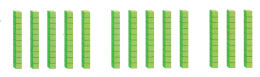___________ tens = ___________
___________ hundred __________ ten
___________ + ___________
13 tens=130
1 hundred 30 ten
100+30
Explanation:The number of blocks in each stack=10
The number of stacks in the row=100
10 tens=100
The number of stacks extra=3
3 tens=30
Total: 100+30=130
We can write numbers in different ways such as place value, expanded form, word form and standard form:
The standard form of 13 tens is 130
The expanded form of 130 is 100+30+0
The word form of 130 is one hundred thirty.
The place value of 130 is 0 is in one’s place, 3 is in the tens place, 1 is in the hundreds place.

Question 3.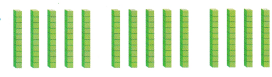___________ tens = ___________
___________ hundred __________ ten
___________ + ___________
14 tens=140
1 hundred 40 tens
100+40=140
Explanation:The number of blocks in each stack=10
The number of stacks in the row=100
10 tens=100
The number of stacks extra=4
4 tens=40
Total: 100+40=140
We can write numbers in different ways such as place value, expanded form, word form and standard form:
The standard form of 14 tens is 140
The expanded form of 140 is 100+40+0
The word form of 140 is one hundred forty.
The place value of 140 is 0 is in one’s place, 4 is in the tens place, 1 is in the hundreds place.

Problem Solving

Circle tens to make 1 hundred. Write the number in different ways.

Question 4.___________ tens = ___________
___________ hundred __________ ten
___________ + ___________Explanation:
17 tens=170
1 hundred 70 tens
100+70=170
The number of blocks in each stack=10
The number of stacks in the first row=100
10 tens=100
The number of stacks in the second row=7
7 tens=70
Total: 100+70=170
We can write numbers in different ways such as place value, expanded form, word form and standard form:
The standard form of 17 tens is 170
The expanded form of 170 is 100+70+0
The word form of 170 is one hundred seventy.
The place value of 170 is 0 is in one’s place, 7 is in the tens place, 1 is in the hundreds place.

Question 5.___________ tens = ___________
___________ hundred __________ ten
___________ + ___________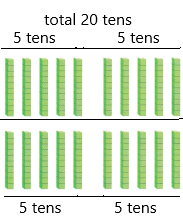Explanation:
20 tens=200
2 hundred 0 tens
200+0=200.
The number of tens in each stack=10
The number of stacks in the first row=100
10 tens=100
The number of stacks in the second row=100
Total:100+100=200
We can write numbers in different ways such as place value, expanded form, word form and standard form:
The standard form of 20 tens is 200
The expanded form of 200 is 200+0+0
The word form of 200 is two hundred.
The place value of 200 is 0 is in one’s place, 0 is in the tens place, 2 is in hundreds place.

Solve. Write or draw to explain.

Question 6.
H.O.T. Kendra has 20 stickers. 10 stickers fill a page. How many pages can she fill?__________ pages
Explanation:
The total number of stickers Ed has=20
The number of stickers she can fill in each page=10
The number of pages she can fill=X
X=20/10
X=2
Therefore, 2 pages she can fill with 20 stickers.

Question 7.
H.O.T. Multi-Step Ed has 150 marbles. How many bags of 10 marbles does he need to get so that he will have 200 marbles in all?____________ bags of 10 marbles
Answer: 5 bags of 10 marbles.
Explanation:
The number of marbles Ed has=150
The number of marbles in each bag=10
The number of marbles needed extra so that he can have 200 marbles=X
The number of bags extra needed extra so that he can have 200 marbles=Y
First of all, calculate the X value.
X=200-150
X=50
Therefore, 50 marbles extra needed to get 200 marbles.
Now calculate the Y value. To know the bags we need to divide X value and marbles in each bag.
Y=50/10
Y=5
Therefore, 5 bags are neede.

Question 8.
Apply A bakery tray that holds 10 muffins. How many muffins will fit on 18 trays?(A) 100
(B) 180
(C) 80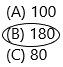Explanation:
The number of muffins that hold a bakery tray=10
The number of muffins will fit in 18 trays=X
Apply cross multiplication:
For each tray, 10 muffins will be there. For 18 trays, we need to calculate.Therefore, In 18 trays, 180 muffins can fit.

Question 9.
Connect Circle tens to make 1 hundred. Write the number in different ways.___________ tens = ___________
___________ hundred __________ ten
___________ + ___________I circled 10 tens into one box which is 100 blocks.
We can write numbers in different ways such as expanded form, standard form, word form, place value.
The standard of 10 tens=100
Place value of 10 tens: 1 hundred 0 ten
The expanded from of 10 tens :100+0+0

Question 10.
TEXAS Test Prep Which has the same value as 17 tens?
(A) 17 hundred
(B) 1 ten 7 ones
(C) 1 hundred 7 tensExplanation: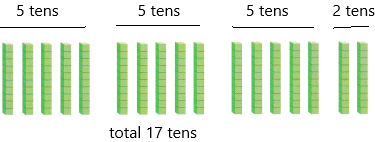This is one way of representing a number.
In the place value chart, we represent this.
17 tens are nothing but 170
The number 170 we can write a standard form, expanded form, word form, and place value.
The standard form is 170.
170 we can write as in place value:
0 is in one’s place
7 is in the tens place
1 is in the hundreds place
The expanded form of a number is 100+70+0

TAKE HOME ACTIVITY • Hove your child draw 110 Xs by drawing 11 groups of 10 Xs.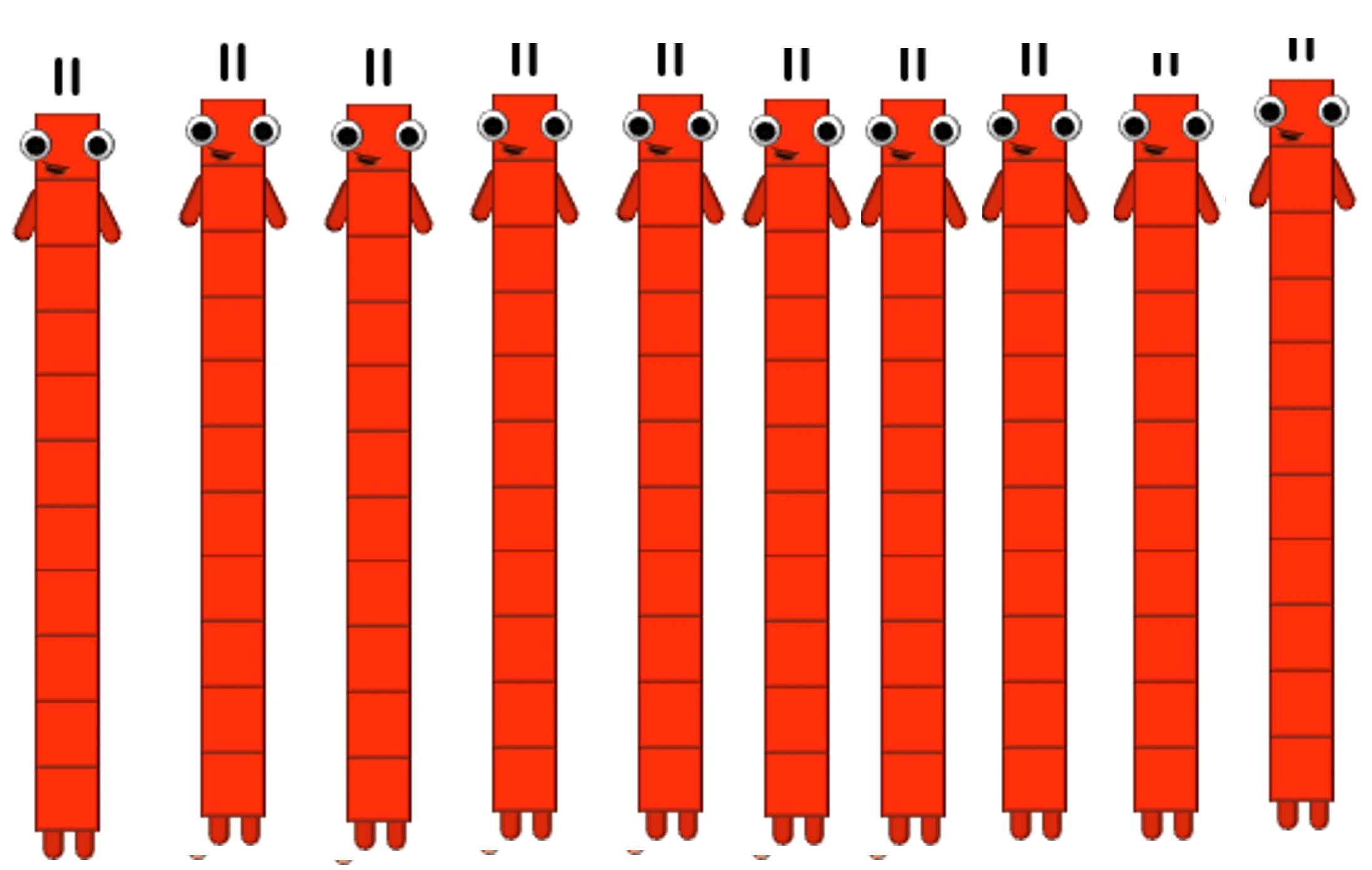In each group, 11 blocks are there.
Make your child draw blocks according to the question.
11*10=110
Therefore, 110 blocks.

### Texas Go Math Grade 2 Lesson 1.3 Homework and Practice Answer Key

Circle tens to make 1 hundred. Write the number in different ways.

Question 1.___________ tens = ___________
___________ hundred __________ ten
___________ + ___________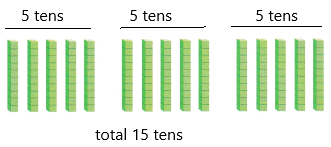Explanation:
The standard form of a number 150
The expanded form of a number is 100+50+0
The word form of a number is one hundred fifty.

Question 2.___________ tens = ___________
___________ hundred __________ ten
___________ + ___________Explanation:
Any number that can be written as the decimal number, between the numbers 1.0 and 10.0, then multiplied by the power of the number 10, is known to be in the standard form.
The number 190 can be written as 1.9×10^2.
In the United States and the countries using the US conventions, the standard form is the usual way of writing numbers in the decimal notation.
The standard of a number is 190
The expanded form of a number is 100+90+0
The word form of a number is one hundred ninety.

Problem Solving

Solve. Write or draw to explain.

Question 3.
Kate has 110 bows. 10 bows fit in a bag. How many bags can she fill?
_________ bags
Explanation:
The number of bows Kate has=110
The number of bows fits in each bag=10
The number of bags she can fill=X
Divide the total bows and bows fits in each bag
X=110/10
X=11
Therefore, 11 bags she can fill.

Question 4.
Multi-Step Dan has 140 markers. How many more boxes of 10 markers does he need to get so that he will have 180 markers in all?
___________ boxes of markers
Explanation:
The number of markers Dan has=140
The number of markers in each box=10
The number of markers is needed so that he will get 180 markers=X
The number of boxes needed to get=Y
first, calculate the X value.
X=180-140
X=40
Therefore, 40 markers are needed more to get 180 markers.
Now to calculate the Y value we need to divide X value and markers in each box.
Y=40/10
Y=4
Therefore, 4 boxes of markers are needed so that he will get 180 markers.

Lesson Check

Question 5.
Mr. Brown orders 150 pencils. How many boxes of 10 pencils should he get?
(A) 10
(B) 15
(C) 20Explanation:
The number of pencils he ordered=150
The number of pencils in each box=10
The number of boxes he can get=X
X=150/10
X=15
Therefore, he gets 15 boxes.

Question 6.
A book bin holds 10 books. There are 12 full bins. How many books are there ¡n all?
(A) 100
(B) 20
(C) 120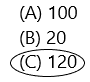Explanation:
The number of books a book bin can hold=10
The number of full bins is there=12
The total number of books are there overall=X
X=12*10
X=120
Therefore, 120 books are there.

Question 7.
Amy orders 250 red ribbons. How many bags of 10 red ribbons will fill the order?
(A) 25
(B) 10
(C) 250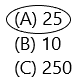Explanation:
The number of red ribbons Amy orders=250
The number of red ribbons needs to fill in each bag=10
The number of bags needed to fill 250 ribbons=X
Now divide to get the answer
X=250/10  (0 and 0 gets cancel, 25/1 is nothing but 25)
X=25
Therefore, 25 bags are needed to fill 250 red ribbons. In each bag, there will be 10 red ribbons.

Question 8.
Multi-Step Saul has 130 baseball cards. How many bags of 10 baseball cards does he need to get so that he will have 200 baseball cards in all?
(A) 7
(B) 70
(C) 60Explanation:
The total number of baseball cards does saul has=130
The number of cards in each bag=10
The number of bags=Y
The number of baseball cards needed to get 200 baseball cards overall=X
Already he has 130 baseball cards so we need to find out the extra cards to get 200 baseball cards. If we subtract cards he has now and overall cards he wants.
X=200-130
X=70.
Therefore, add 70 to the 130 to get the 200 baseball cards.
Thus, 70 cards are needed to get 200 cards overall.
To calculate the number of bags divide X value and cards in each bag.
Y=70/10
Y=7.
Therefore, 7 bags are needed.

Scroll to Top